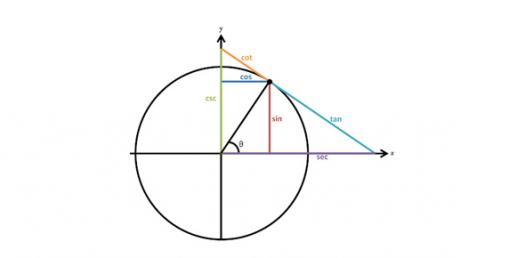# Trigonometric Ratios

12 Questions | Total Attempts: 2089SettingsThis quiz tests students' understanding of the three basic trigonometric ratios of sine, cosine, and tangent. It also includes a few application problems.

• 1.
Find the value of x. Round to the nearest tenth.
• A.

3.5

• B.

12.1

• C.

6.1

• D.

4

• 2.
Find the value of x. Round to the nearest tenth.
• A.

3.3

• B.

3.1

• C.

24.7

• D.

8.5

• 3.
Find the value of x. Round to the nearest tenth.
• A.

6.2 cm

• B.

12.7 cm

• C.

15.5 cm

• D.

10.9 cm

• 4.
Find the value of x. Round to the nearest degree.
• A.

30

• B.

60

• C.

70

• D.

85

• 5.
Find the value of x. Round to the nearest tenth.
• A.

67

• B.

22

• C.

83

• D.

53

• 6.
A large totem pole in the state of Washington is 100 feet tall. At a particular time of day, the totem pole casts a 249 foot long shadow. Find the measure of and A to the nearest degree.
• A.

68

• B.

45

• C.

35

• D.

22

• 7.
Write the ratios for sin X and cos Y.
• A.

• B.

• C.

• D.

• 8.
Find the value of x. Round to the nearest tenth.
• A.

12.5

• B.

10

• C.

13

• D.

9.7

• 9.
Find the value of x. Round to the nearest tenth.
• A.

12.9

• B.

8.5

• C.

12.4

• D.

8.1

• 10.
Find the value of x. Round to the nearest tenth.
• A.

52.6

• B.

52.9

• C.

6.2

• D.

6.5

• 11.
An airplane over the Pacific sights an atoll at an angle of depression of 5 degrees. At this time, the horizontal distance from the airplane to the atoll is 4629 meters. What is the height of the plane to the nearest meter?
• A.

403 m

• B.

405 m

• C.

4611 m

• D.

4647 m

• 12.
To find the height of a pole, a surveyor moves 140 feet away from the base of the pole and then, with a transit 4 feet tall, measures the angle of elevation to the top of the pole to be 44 degrees. To the nearest foot, what is the height of the pole?
• A.

145 ft

• B.

149 ft

• C.

135 ft

• D.

139 ft

Related TopicsBack to top